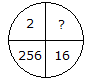# Verbal Reasoning - Character Puzzles - Discussion

### Discussion :: Character Puzzles - Character Puzzles 1 (Q.No.34)

34.

Which one will replace the question mark ?[A]. 8 [B]. 32 [C]. 4 [D]. 16

Explanation:

(16)2 = 256

Therefore, (2)2 = 4.

 Pranay Chauli said: (Jan 3, 2017) 16 ÷ 2 = 8; At the same way 256 ÷ 8 = 32.

 Priyabrata Mohanty said: (Aug 1, 2018) I think answer will be 32.

 Durgesh said: (Aug 4, 2018) Yes, it will be 32.

 Gaurav said: (Sep 1, 2018) 2^(2^0) 2^(2^1) 2^(2^2) 2^(2^3). Therefore, the correct answer is 4.

 Aadi said: (Nov 2, 2018) 2 * 8 = 16 32 * 8= 256 (256 ÷ 8=32). Ans is 32.

 Bhavya Bankeraika said: (Sep 2, 2020) The answer should be 32.